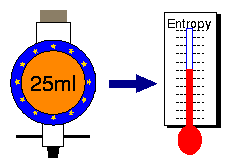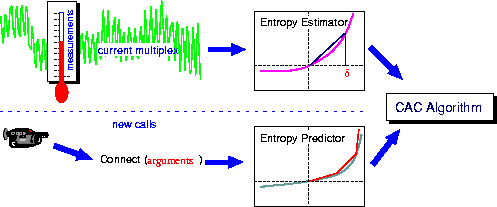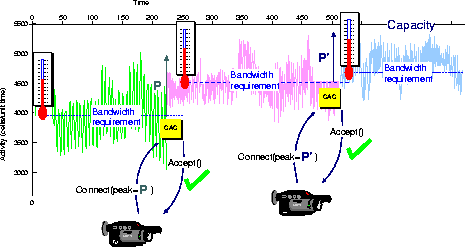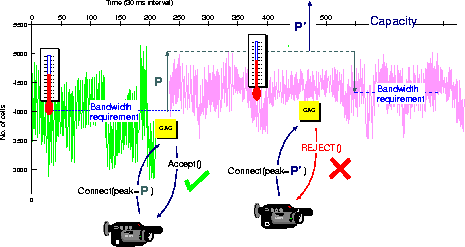The Measure Project"On-line Measurement of Resource Management"
 The Measure CAC Algorithm Measure NetOS
 The following diagrams illustrate the Measure Connection Admission Control (CAC) algorithm. The traditional approach is for connections to describe their resource requirements and QoS parameters to the CAC, which admits calls based on either complex and difficult to determine parameters or on the peak rate requirements. The use of on-line measurements and estimation for admission control is extremely appealing because of its simplicity and the ability to deal with sources that are not accurately characterised. In the Measure CAC algorithms parameters supplied by a new call request are passed to the predictor which attempts to predict the SCGF, and thence the bandwidth requirement, of the new call. This is combined with the estimated bandwidth requirement of the current multiplex, produced by the estimator, to decide whether or not to admit the call.The above diagrams show the behaviour of the Measure CAC algorithm. Given a current multiplex of calls, the system estimates the bandwidth requirement of the multiplex from the estimated SCGF using the entropy estimator (depicted here by a thermometer). The predictor used in the Measure algorithm requires a new call attempt to declare only its peak rate P. The predictor is pessimistic; it assumes that the incoming call has a bandwidth requirement equal to P. The CAC algorithm sums the peak rate P, and the estimated bandwidth requirement of the multiplex; if the total is less than the link capacity, then the connection can be accepted without violating the QoS of any calls, as in the second diagram. As soon as the new call commences, the estimator uses measurements of the new multiplex to revise its estimate of the current bandwidth requirement. When the next call attempt arrives, the procedure is repeated, as shown. If a new call attempt arrives before the algorithm has developed an accurate estimate of the new bandwidth requirement, as shown in the third diagram, the algorithm acts conservatively. It uses the most recent stable estimate of the bandwidth requirement, plus the sum of the peak rates of all subsequently admitted calls. Thus, in the this case, the second call is rejected, because the sum of the two peaks P + P' and the first bandwidth requirement estimate exceeds the link capacity.